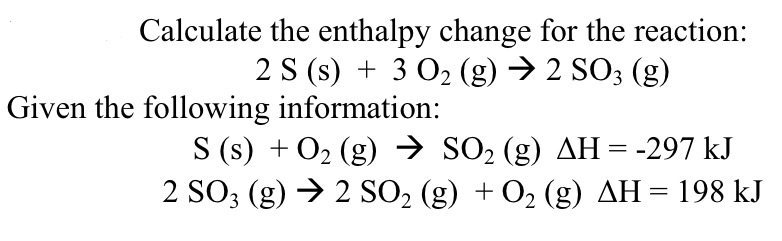Home / Answered Questions / Other / calculate-the-enthalpy-change-for-the-reaction-2-s-s-3-o2-g-2-so3-g-given-the-following-information--aw543

# (Solved): Calculate The Enthalpy Change For The Reaction: 2 S (s) + 3 O2 (g) â†’ 2 SO3 (g) Given The Following...Calculate the enthalpy change for the reaction: 2 S (s) + 3 O2 (g) â†’ 2 SO3 (g) Given the following information: S (s) + O2 (g) â†’ SO2 (g) AH = -297 kJ 2 SO3 (g) â†’ 2 SO2 (g) + O2 (g) AH = 198 kJ

We have an Answer from Expert